# Introducing d3-scale

I’d like D3 to become the standard library of data visualization: not just a tool you use directly to visualize data by writing code, but also a suite of tools that underpin more powerful software.

To this end, D3 espouses abstractions that are useful for any visualization application and rejects the tyranny of charts.

As Leland Wilkinson wrote in The Grammar of Graphics,

If we endeavor to develop a charting instead of a graphing program, we will accomplish two things. First, we inevitably will offer fewer charts than people want. Second, our package will have no deep structure. Our computer program will be unnecessarily complex, because we will fail to reuse objects or routines that function similarly in different charts. And we will have no way to add new charts to our system without generating complex new code. Elegant design requires us to think about a theory of graphics, not charts.

If visualization is constructing “visual representations of abstract data to amplify cognition”, then perhaps the most important concept in D3 is the scale, which maps a dimension of abstract data to a visual variable.

And now scales are available in a standalone library, d3-scale.

But what is a “dimension” of data? Or a “visual variable”? Consider a table of data, as in a spreadsheet. Each row in the table is a vector, and each column is a dimension. A dimension is just a named attribute whose values have a particular meaning, such as a price in dollars.

We typically think of dimensions as spatial and quantitative, such as a position in space represented by real numbers ⟨x, y, z⟩. Yet with abstract data there are also non-quantitative dimensions; for example, diamond cut quality (fair, good, very good, ideal) is ordinal, while diamond cut shape (princess, round, marquise, etc.) is categorical.

Visual variables are best explained by Jacques Bertin in Semiology of Graphics. He described how graphical marks (say, dots in a scatterplot) can represent data using planar position ⟨x, y⟩ and a luminous dimension z:

Within the plane a mark can be at the top or the bottom, to the right or the left. The eye perceives two independent dimensions along X and Y, which are distinguished orthogonally. A variation in light energy produces a third dimension in Z, which is independent of X and Y…
The eye is sensitive, along the Z dimension, to 6 independent visual variables, which can be superimposed on the planar figures: the size of the marks, their value, texture, color, orientation, and shape. They can represent differences (≠), similarities (≡), a quantified order (Q), or a nonquantified order (O), and can express groups, hierarchies, or vertical movements.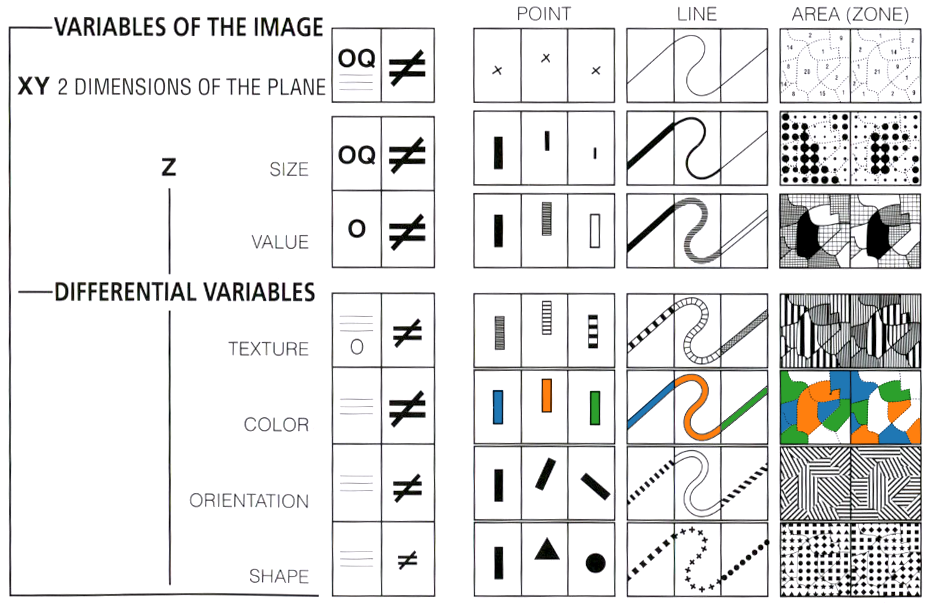From Semiology of Graphics, colorized by the author.

Thus, a scale is a function that takes an abstract value of data, such as the mass of a diamond in carats, and returns a visual value such as the horizontal position of a dot in pixels. With two scales (one each for x and y), we have the basis for a scatterplot.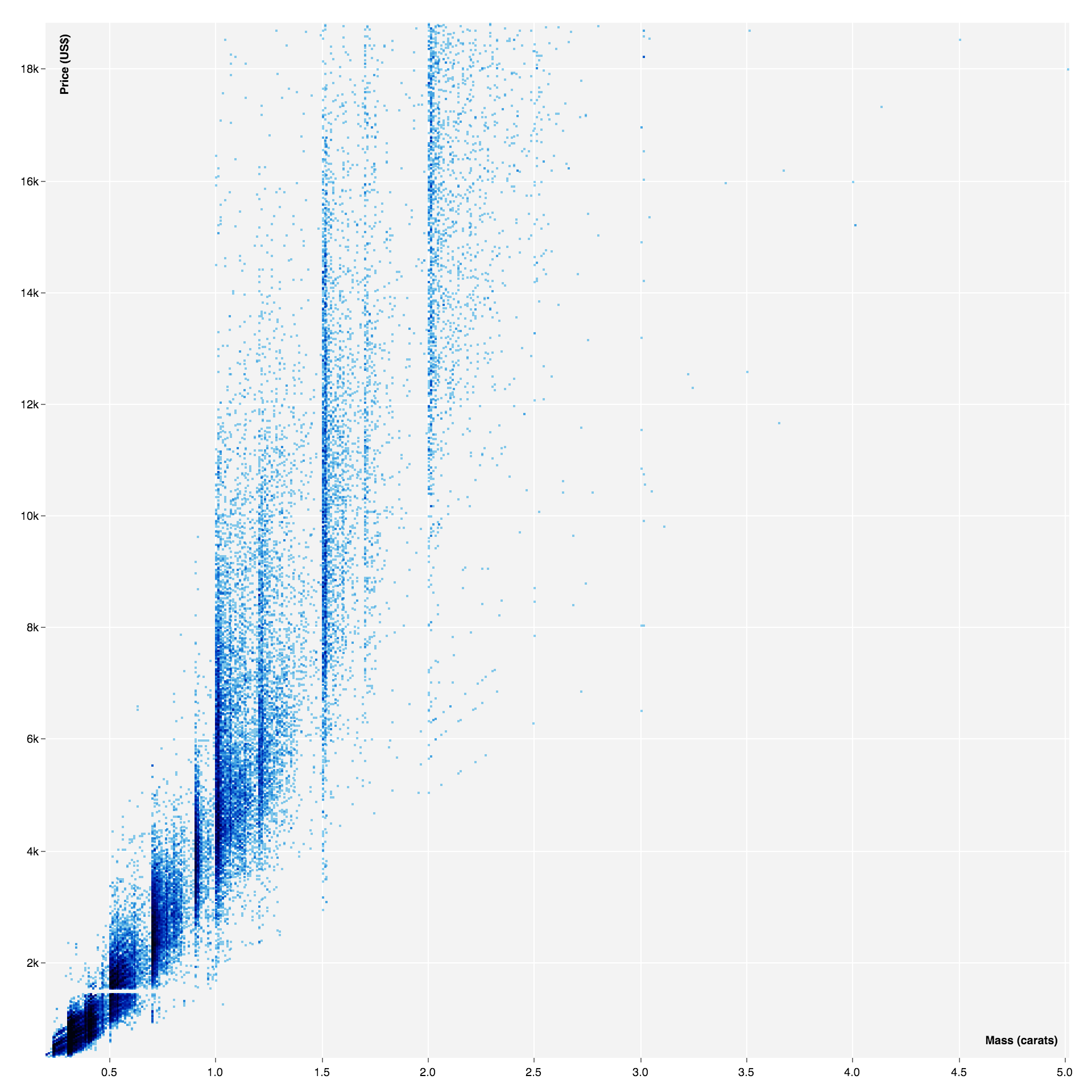The relationship between diamond mass and price. View source.

To illustrate how scales work, imagine how you might compute x and y for each dot above. Given some values derived from data (minCarat, maxCarat, minPrice, maxPrice) and some from the chart size (width, height), you might do something like this:

`function x(carat) {  return (carat - minCarat)      / (maxCarat - minCarat)      * width;}`
`function y(price) {  return height      - (price - minPrice)      / (maxPrice - minPrice)      * height;}`

The lightest diamond is placed at the chart’s left edge, the heaviest diamond is placed at the chart’s right edge, and so on. Note that the range of the y-scale is inverted because graphics systems put the origin in the top-left corner whereas scatterplots put it in the bottom-left.

Like the above, D3’s quantitative scales are functions configured by two intervals. The input domain is an interval in the abstract dimension of data, often the extent of the observed values. The output range is an interval in the visual variable, such as the visible area defined by the chart size.

`var x = d3.scaleLinear()    .domain(d3.extent(data, function(d) { return d.carat; }))    .range([0, width]);`
`var y = d3.scaleLinear()    .domain(d3.extent(data, function(d) { return d.price; }))    .range([height, 0]);`

But scales do much more than basic arithmetic!

For one, it is now trivial to apply quantitative transformations: replace a linear scale with a logarithmic or power scale. A linear scale is a good default choice because it preserves proportionality, but a log or pow scale may aid the differentiation of data that is not uniformly distributed. (Log scales are also good for showing change.)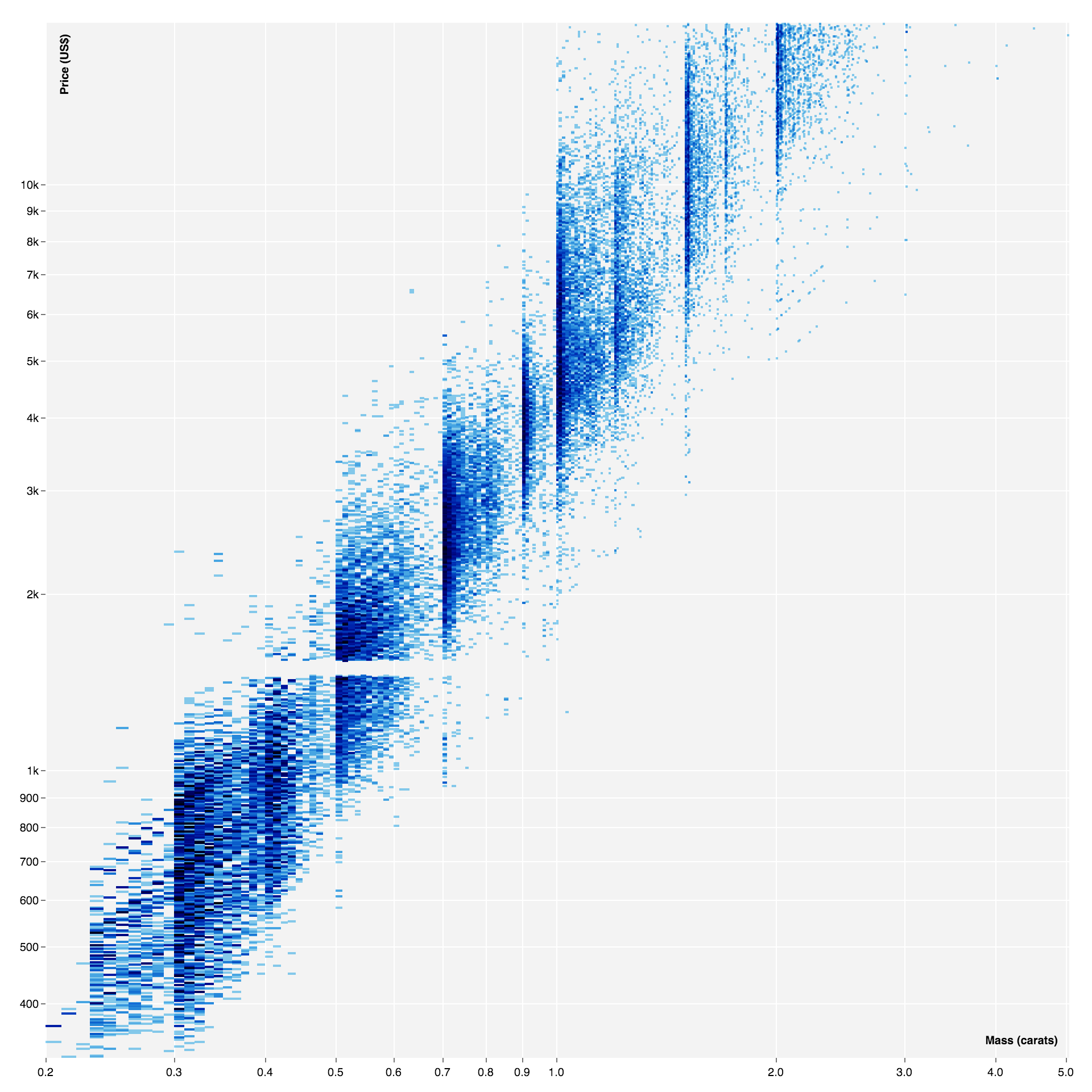The previous scatterplot modified to use log scales. View source.

For two, scales alleviate the tedium of drawing legible axes by generating and formatting nice, round values (ticks) from the domain. A scale’s ticks are type-appropriate: for example, the log ticks above are uniformly-spaced within each power of ten, while a time scale uses calendar intervals.

Most scales are bidirectional: you can invert the mapping from visual representation back to abstract data, facilitating interaction. For example, a brushed interval in pixels can be inverted to abstract data for querying.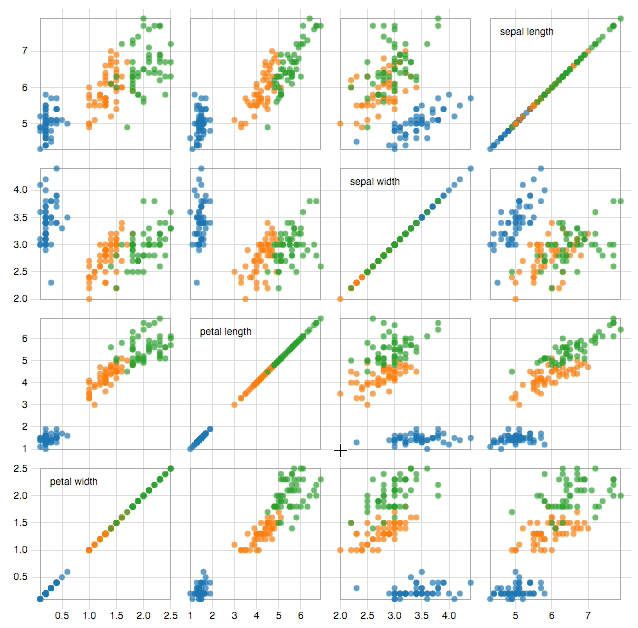Brushing a scatterplot matrix. View source.

And there are scales for ordinal and categorical data. The band scale, for instance, simplifies the calculation of bar widths and positions, allowing configurable padding, alignment and rounding.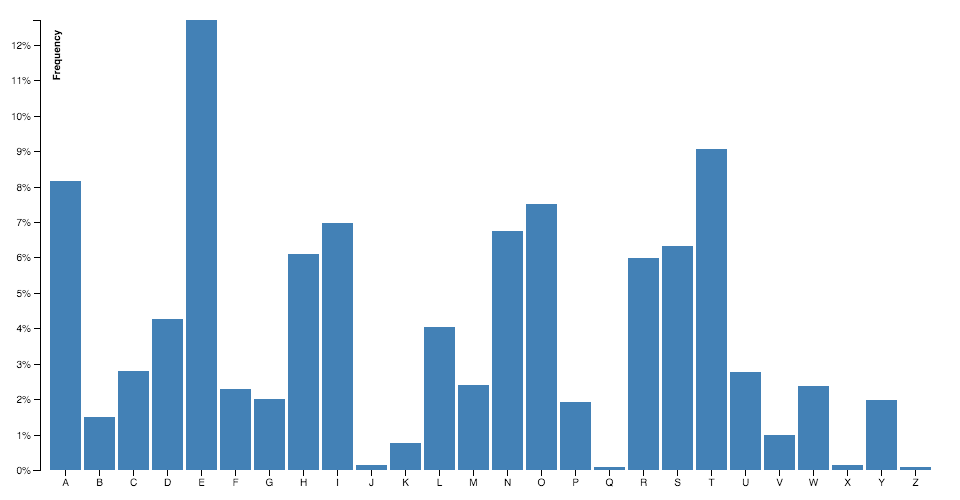The frequency of English letters. View source.

Yet scales are not just for positioning; they are for computing any visual variable. Scales can interpolate symbol sizes, font sizes, stroke widths, colors in various color spaces, geometric transforms, shapes and even deeply-nested objects. Below, a scale represents quantity using angular orientation, with small numbers leaning left (\) and large numbers leaning right (/). This reveals the behavior of a sorting algorithm on an array of numbers: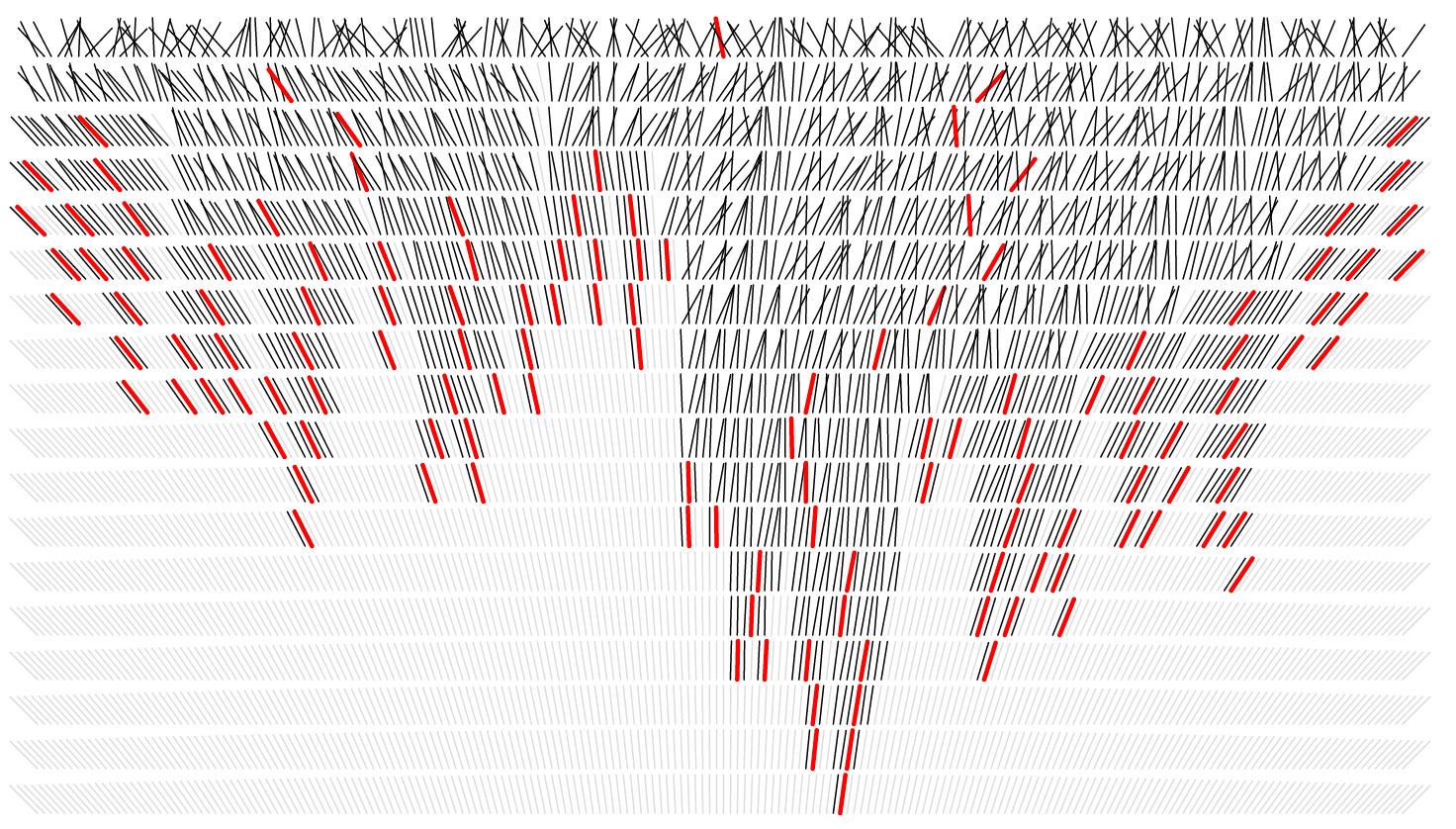Visualizing quicksort. From Visualizing Algorithms.

Below, a square-root scale computes the appropriate radius so that the area of each county’s bubble is proportional to the number of people living there: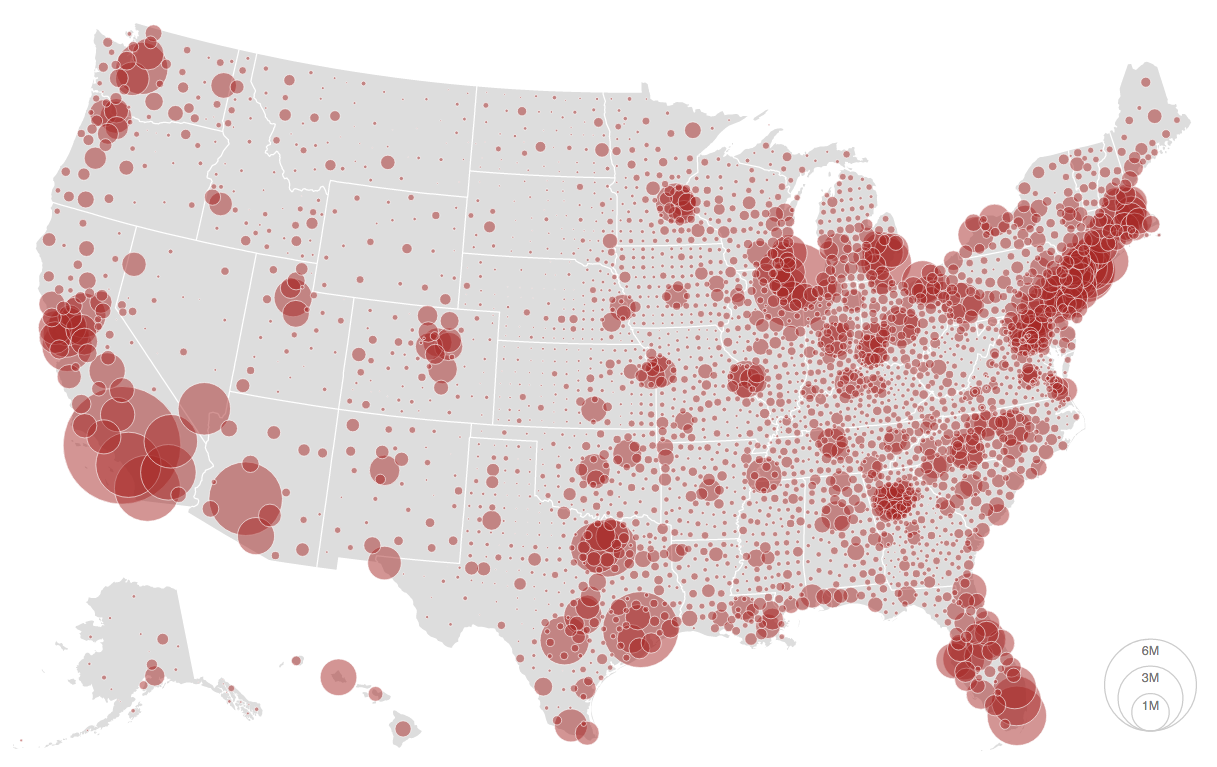Population in 2008. From Let’s Make a Bubble Map.

Below, a comparison of perceptually-uniform sequential color scales used for a choropleth of unemployment rate: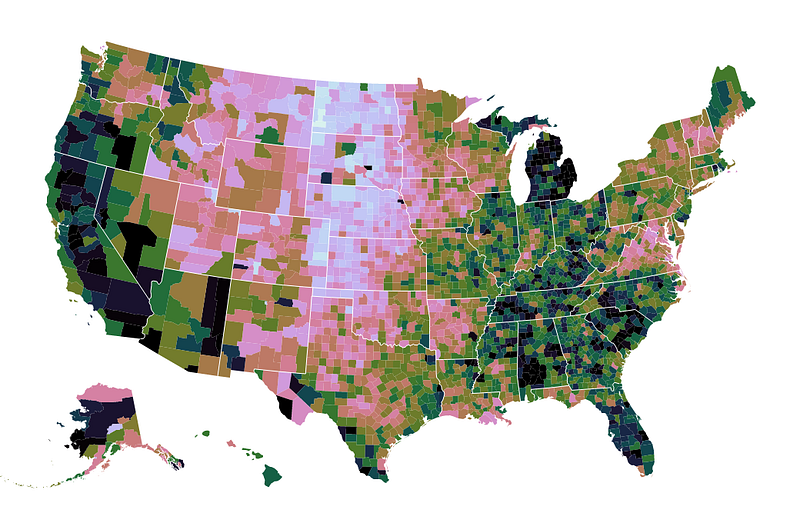Unemployment in 2008, using magma, viridis and cubehelix. Darker colors indicate a higher unemployment rate. View source.

You can even create piecewise scales for diverging colors, or quantize scales for applying discrete breaks to continuous data.

So, try it out! And check out the other new D3 modules, too, such as d3-time, d3-format, and d3-shape.

Happy scaling!